Physical Properties of Gaseous State

• Gases have no definite volume and they do not have specific shape.
• Gases mix evenly and completely in all proportions without any mechanical aid.
• Their density is much lower than solids and liquids.
• They are highly compressible and exert pressure equally in all directions.

## Boyle’s law (Pressure-Volume Relationship)

At constant temperature, the volume of a given mass of gas is inversely proportional to its pressure.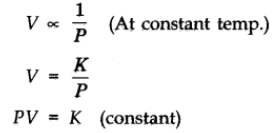Here, V is the volume, P is the pressure.

K = Constant of proportionality.

The value of constant k, depends upon the amount of gas, the temperature of the gas and the units in which P and V are expressed.

Let V1 be the volume of the gas at a pressure P1. By keeping the temperature constant, if the pressure is increased to P2 then the volume will decrease to V2. According to the law,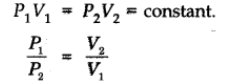Isotherms: Graphs of V vs p or pV vs p at constant temperature known as Isotherms.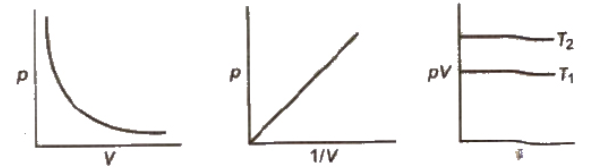## Charles’ Law (Temperature-Volume Relationship)

The volume of the given mass of a gas increases or decrease by 1/273 of its volume for each degree rise or fall of temperature respectively at constant pressure.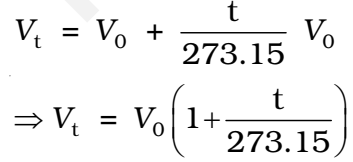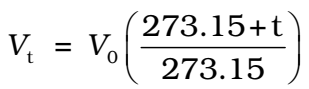The volume of a given mass of a gas is directly proportional to the absolute temperature at constant pressure.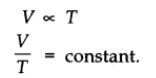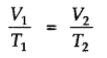Absolute zero is the theoretically possible temperature at which the volume of the gas becomes zero. It is equal to 00C or 273.15K.

Isobars: A graph of V vs T at constant pressure is known as isobar.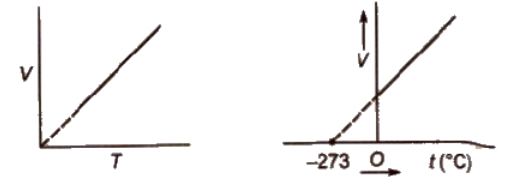Charles’ law explains that gases expand on heating, so hot air is less dense than cold air.

## Gay Lussacs’ Law (Pressure-Temperature Relationship)

At constant volume, pressure of a given mass of a gas is directly proportional to the temperature.

Mathematically,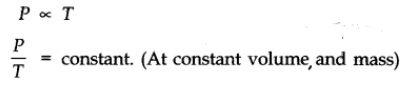Pressure Vs. temperature (Kelvin) graph at constant molar volume is shown in figure.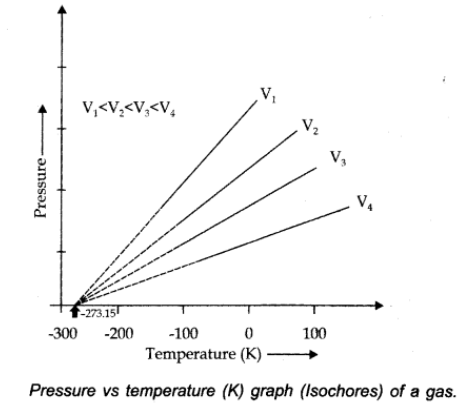Isochore: Each line of graph is called isochore.